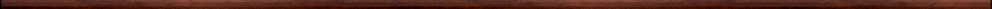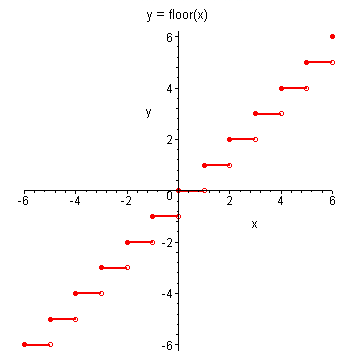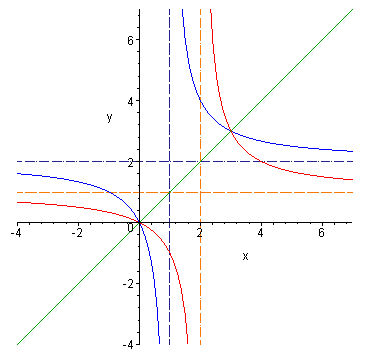Maple worksheets on functionsPrecalculus topics:

They are all compatible with Classic Worksheet Maple 10.Graphs of functions - graphs.mws

• Graphs of common functions.
• Translating graphs
• Powers of x

Sets of numbers and the domain and range of a function - domrng.mws

• Sets and set operations.
• Sets and set operations with Maple.
• Rational, irrational and real numbers.
• Interval notation.
• The domain and range of a function.

Cubic curves - cubic.mws

• Curves of the form y = a*x^3.
• Translations of y = a*x^3.
• The curve y = x^3- x obtained by a sheering transformation.
• Curves of the form y = x^3 + a*x.
• Curves of the form y = x^3 + a*x + b.

Cubic curves II - cubic2.mws

• The solution of the cubic equation x^3 + a*x + b = 0 (3 real roots).
• The case of "equal roots".
• The turning points on y = x^3 + a*x.
• The local maximum point as a limit.
• The solution of the cubic equation x^3 + a*x + b = 0 (1 real root).
• Cubic curves of the form x^3 + p*x^2 + q*x + r.

Composition of functions - composition.mws

• Composition of functions in Maple.
• More examples
• Repeated composition and the logistic function.

Piecewise functions etc. - piecewise.mws

• Functions defined in pieces using piecewise.
• Functions defined in pieces using proc.
• Even and odd functions.
• The integer and fractional parts of a number.

Inverse functions - invfcns.mws

• One-to-one functions.
• The inverse of a one-to-one function.
• Finding inverse functions.
• Graphs of inverse functions.

Self-inverse functions - selfinv.mws

• The composition of two one-to-one functions and its inverse.
• What is a self-inverse function?
• A way to construct examples of self-inverse functions.Top of page

Main index